# Plus-construction

Jump to: navigation, search

Quillen plus-construction

A mapping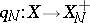between spaces of the homotopy type of connected CW-complexes (cf. also CW-complex), which has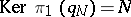(necessarily a perfect normal subgroup of) and is an acyclic mapping. This means thatsatisfies the following, equivalent, conditions:

the homotopy fibre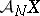of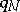is acyclic;induces an isomorphism of integral homology and a trivial action of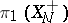on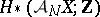;induces an isomorphism of homology with any local coefficient system of Abelian groups;

ifhas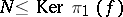, then there is a mapping, unique up to homotopy, such that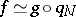.

Whenis always chosen to be the maximum perfect subgroup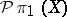of the fundamental group of the domain, and the mapping is taken to be a cofibration (in fact, it can be taken to be an inclusion in a space formed by the adjunction of 2- and 3-cells), this determines a functor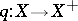. General references are [a6], [a1]. A fibre sequence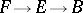induces a fibre sequenceif and only if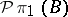acts on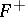by mappings freely homotopic to the identity; when the spaceis nilpotent, this condition reduces toacting trivially on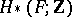[a2].

The construction, first used in [a10], was developed by D. Quillen [a15] in order to define the higher algebraic-theory of a ringas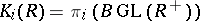, where the infinite general linear group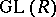is the direct limit of the finite-dimensional groups, and the plus-construction is applied to its classifying spaceto obtain an infinite loop space (hence spectrum) [a16]. General references are [a12], [a1]. Reconciliation with other approaches to higher-theory is found in [a5], [a13]. Subsequently, similar procedures have been employed for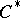-algebras [a8] and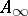ring spaces [a4].

Every connected space can be obtained by the plus-construction on the classifying space of a discrete group [a9]. Thus, the construction has also been studied for its effect on the classifying spaces of other groups, for example in connection with knot theory [a14] and finite group theory [a11]. Relations with surgery theory can be found in [a7]. For links to localization theory in algebraic topology, see [a3].